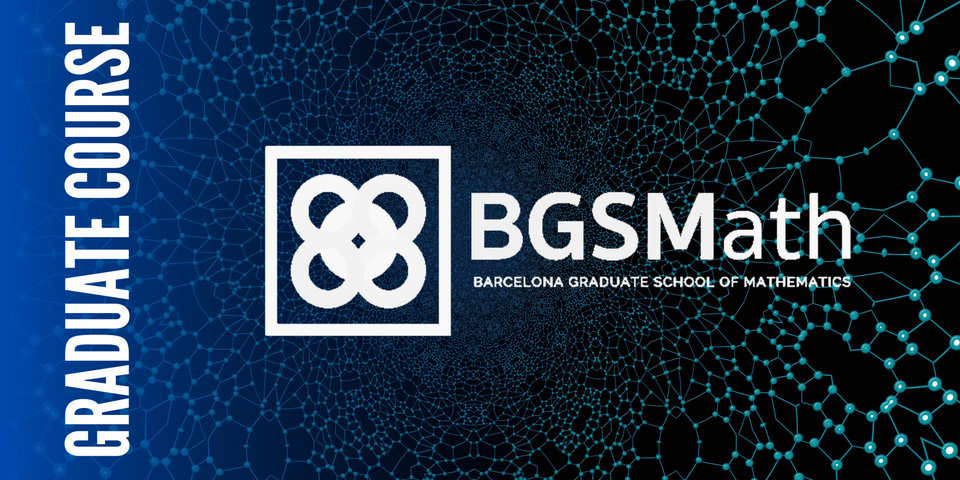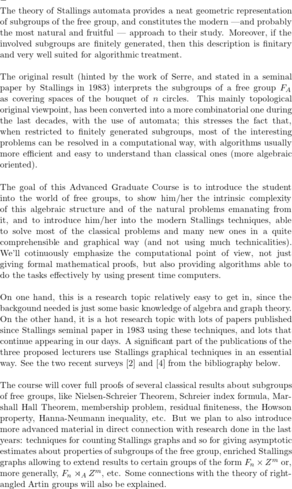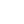Select Page# BGSMATH: Stallings Automata and Applications

Advanced course / School
From January 17, 2023
to February 16, 2023

Schedule:

17th January - 16th Februrary

Tuesdays and Thursdays, from 16h to 18h.

Registration deadline 10 / 01 / 2023

course descriptionlecturers

Enric Ventura | UPC
Jordi Delgado | UPC
Pascal Weil | Laboratoire bordelais de recherche en informatique (LaBRI), Université Bordeaux I

organizer

#### SYLLABUS

Introduction: Free group, automata, algorithmic problems (2h). Here we’ll start with the categorical definition of free group, its construction as the group of words over an alphabet modulo cancellation, and its first algebraic properties. We’ll also introduce the basic concepts and notation from automata and graph theory.

Automata and languages (2h). Here we’ll set up the connection between automata (i.e., graphs with certain decorations), languages, and the free group. And we’ll prove few technical lemmas closely relating these two central aspects of the theory.

The Stallings bijection (2h). Here we’ll establish the details of the central result in Stallings theory: there is a bijection between a certain king of automata and the lattice of subgroups of the free group. Moreover, we’ll stress the computability of the two maps when restricted to finitely generated subgroups and finite graphs, respectively. As a first corollary, we get Nielsen-Schreier Theorem almost with no extra effort.

First applications (2h). This sesion will be devoted to the first algebraic applications: membership problem (including explicit rewritting in terms of the given generators), finding a basis of a subgroup, conjugacy problem for subgroups. We’ll stress some similarities and some (big) differences between the properties of the lattice of subgroups of the free group, and those of the (much more classical) lattice of subspaces of a vector space.

Index and Marshall Hall Theorem (2h). Here, we’ll explain the relation between vertices of the automata and cosets of the subgroup. We’ll obtain a graphical characterization for finite index, and the Schreier index formula relating index and rank. As a corollary we obtain also a proof of Marshall Hall Theorem, and the residual finiteness of free groups.

Interseccions of subgroups (2h). Here, we’ll introduce the pull-back technique for automata, and see the relation with intersections of subgroups. This will allow us to prove the Howson property for free groups (intersections of finitely generated subgroups are always finitely generated), and the Hanna-Neumann inequality (removing the coefficient 2 has been a famous problem solved recently with the help of other techniques out of the scope of this course). On the way, we’ll be able to compute a basis for the intersection of two subgroups (a problem which seems quite tricky at a first look). With no more effort, the same techniques allow to compute intersections of cosets, and to understand malnormality.

Counting automata and counting subgroups (2h). In this sesion we’ll introduce a recent technique developped to count (or asymptotically estimate) the amount of subgroups satisfying certain properties. This is done by counting automata, which consists on counting partial injections (they form an inverse monoid generalizing the classical symmetric group in an interesting way).

Enriched Stallings automata I (2h). This sesion will be dedicated to explain a recent extension of Stallings automata: by decorating the automata with integral vectors in an appropriate way, we obtain a bijection (similar to the Stallings one) between a certain kind of enriched automata and the lattice of subgroups of $F_n \times Z^m$, the direct product of a free and a free abelian group. Of course, the algebraic questions related to the free abelian part usually become systems of equations, but the interplay between the two parts is interesting and sometimes highly non-trivial.

Enriched Stallings automata II: intersections (2h). Despite the apparent similarity, the lattices of subgroups of $F_n$ and $F_n \times Z^m$ behave very differently, specially when we consider intersections. A big difference is that the second one does not satisfy the Howson property: it admits pairs of finitely generated subgroups whose intersection is not fnitely generated. By looking carefully at the pull-back of (enriched) Stallings automata, we’ll explain how to decide when is the corresponding intersection finitely generated and when it is not.

Applications to semidirect products and beyond (2h). In this last sesion we’ll give an overview on further aplications of these techniques and its modern developments. One direction are semidirect products $F_n \rtimes_A Z^m$ (given by a set A of invertible integral matrices): these groups are much more complicated than direct products, since there starts appearing some algorithmically unsolvable problems; with less accuracy and more difficulties, one can approach these groups by using enriched Stallings automata whose vectors move arround according to the action matrices fixed in advance. Another direction is right-angled Artin groups (RAAG): the connection is along Droms groups, a special kind of RAAG’s which are accesible from free groups by iteratively taking finitely many direct products with $Z^m$, and finitely many free products. Several algoritmnic properties can be extended from $F_n \times Z^m$ to Droms groups.

#### BIBLIOGRAPHY

1. L. Bartholdi and P.V. Silva, “Rational subsets of groups”, Chapter 23 from J.E. Pin “Handbook on automata theory”, EMS Publishing House (2021), 1608 pages. Available at arXiv:1012.1532.
2. J. Delgado, E. Ventura, “Autòmats de Stallings, un camí d’anada i tornada” (in catalan). To appear at Butlletí de la Societat Catalana de Matemàtiques. Available at https://arxiv.org/pdf/2202.07642.pdf
3. J. Delgado and E.Ventura, “Stallings automata for free-times-abelian groups: intersections and index”. Publicacions Matemàtiques 66 (2022), 789–830.
4. J. Delgado, E. Ventura, “A list of applications of Stallings automata”, Transactions on Combinatorics 11(3) (2022), 181—235. Available at https://arxiv.org/pdf/2109.01268.pdf
5. I. Kapovich and A. Myasnikov. “Stallings foldings and subgroups of free groups”. Journal of Algebra 248.2 (Feb. 2002), pp. 608–668.
6. A. Miasnikov, E. Ventura, and P. Weil. “Algebraic extensions in free groups”. In: Geometric Group Theory. Ed. by G.N. Arzhantseva, J. Burillo, L. Bartholdi, and E.Ventura. Trends in Mathematics. Birkhäuser Basel, Jan. 2007, pp. 225–253.
7. J.R. Stallings. “Topology of finite graphs”. Inventiones Mathematicae 71 (Mar. 1983), pp. 551–565.
8. N.W.M. Touikan, “A fast algorithm for Stallings’ folding process”. International Journal of Algebra and Computation 16(06) (Dec. 2006), pp. 1031–1045.For inquiries about this event please contact the research programs coordinator Ms. Núria Hernández at nhernandez@crm.cat​​